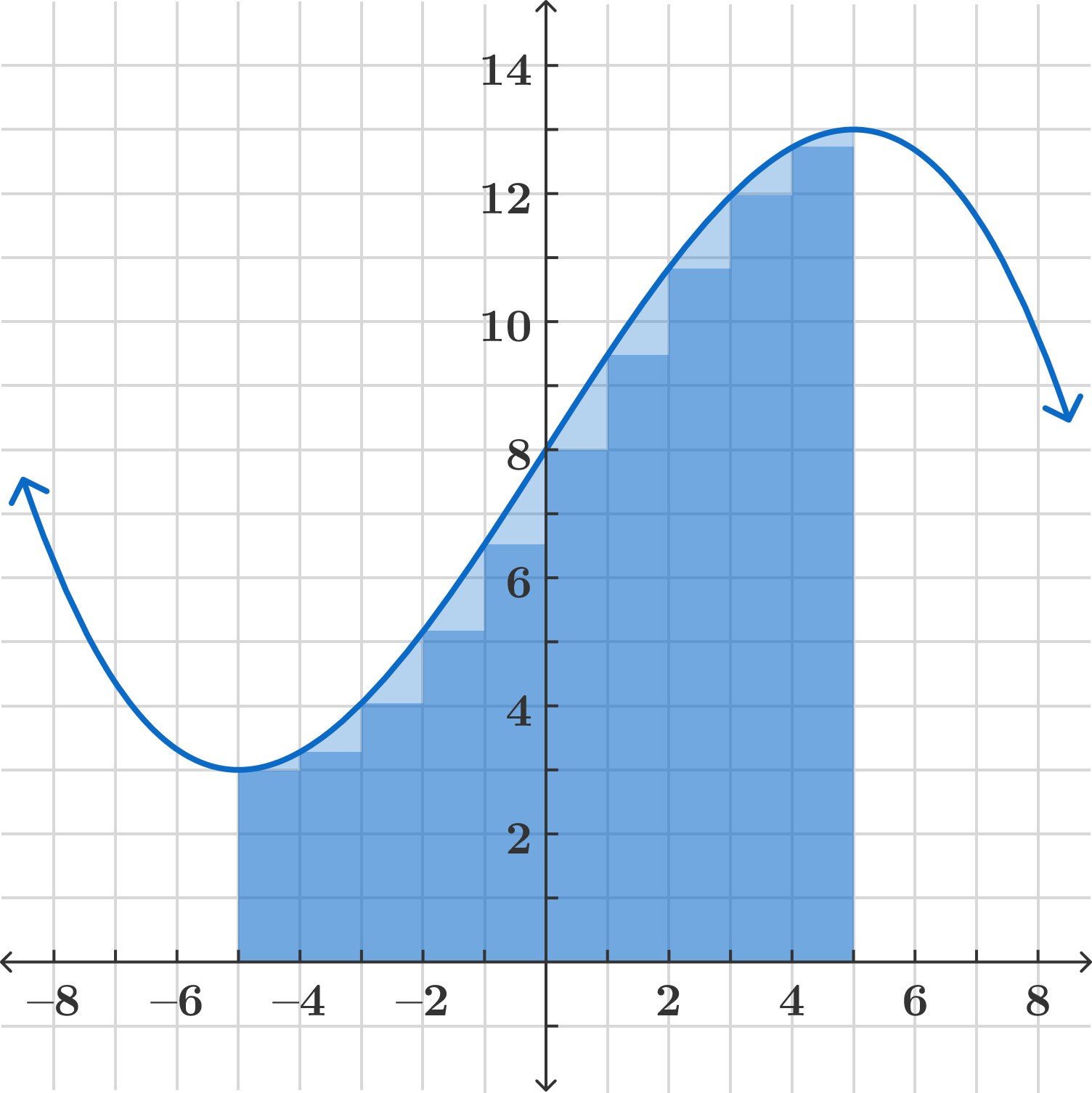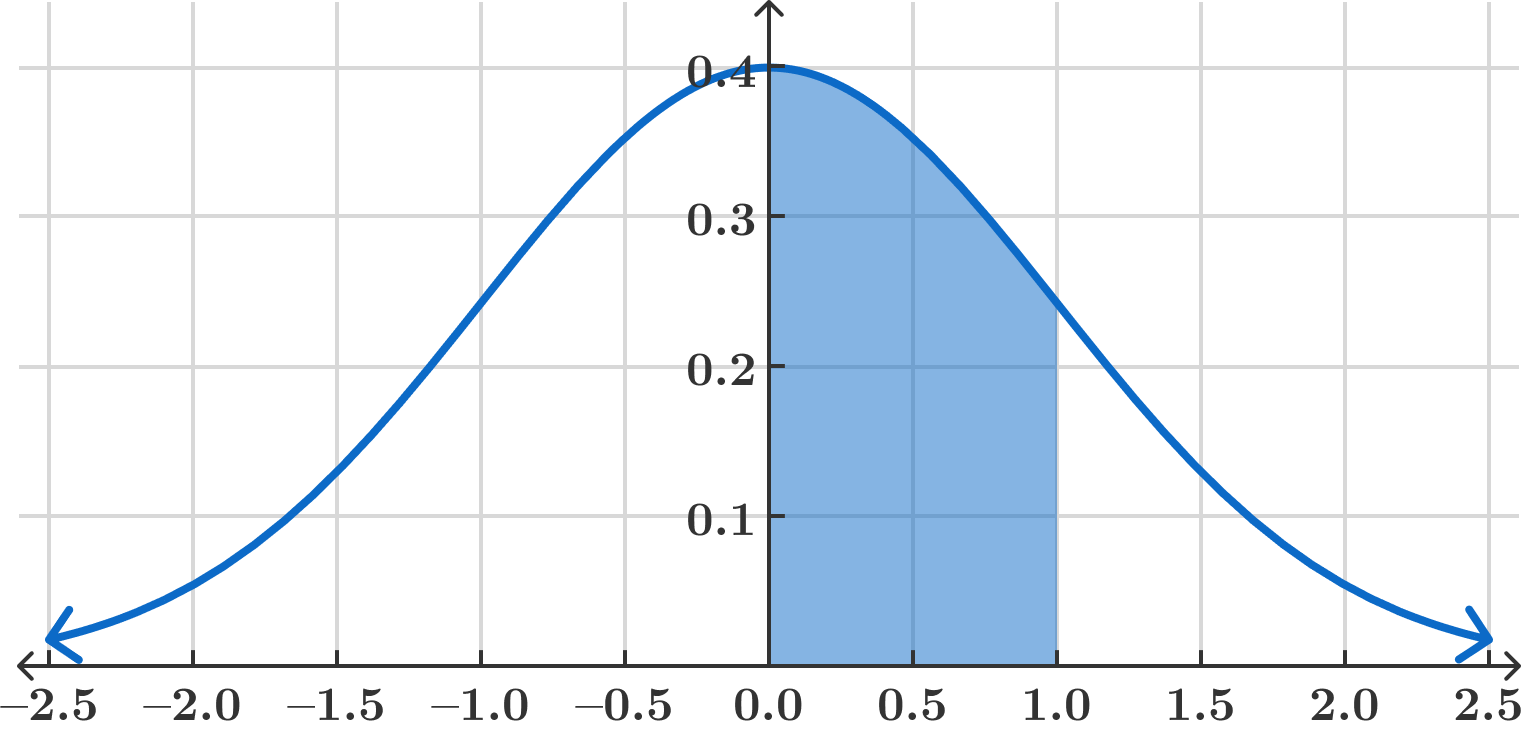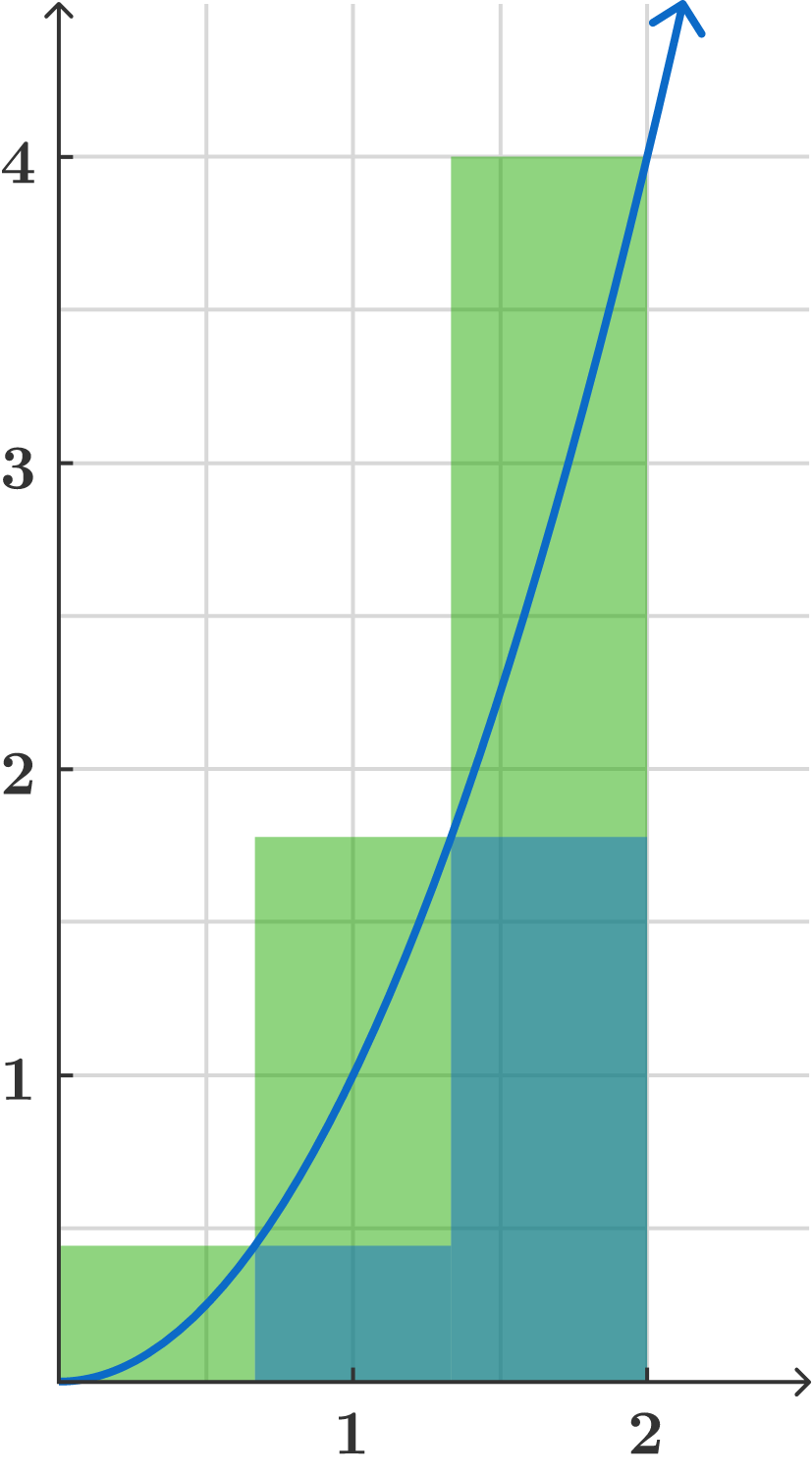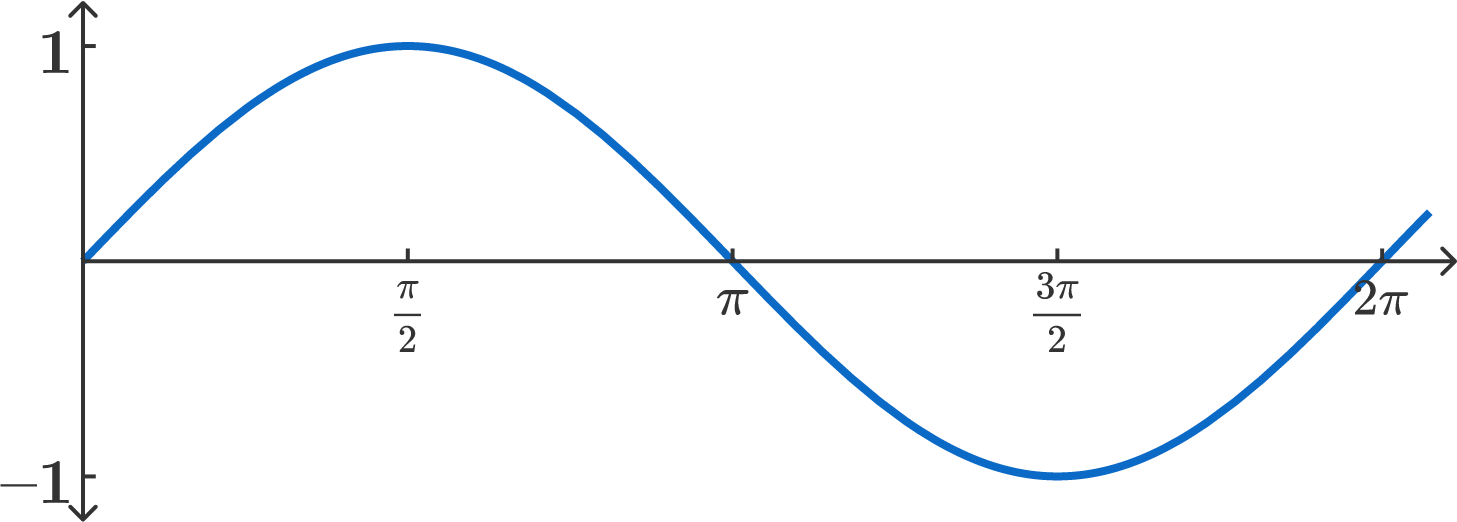### Integral Calculus

We’ve seen that Riemann sums are connected to various natural questions: distance traveled, average value, and continuous probability can all be represented by the limit of a Riemann sum. This probably means they're important — so let's give them a name!

We define the integral as follows:

\begin{aligned} \int_a^b f(x) \; dx &= \lim_{n \rightarrow \infty} \text{RS}(f,a,b,n) \\ &=\lim_{n \rightarrow \infty} \sum_{i=0}^{n-1}f\left(a + i \cdot \frac{b-a}{n}\right) \left(\frac{b-a}{n}\right). \end{aligned}

The notation is supposed to suggest the limit of the Riemann sums: the summation symbol $\sum$ becomes $\int$ (which is a curved "S" for "Sum"), and the interval size $\left(\frac{b-a}{n}\right)$ becomes $dx,$ which represents the length of an infinitely small interval. Also, note that $x$ here is just a dummy variable; we could just as easily write this as $\int_a^b f(t)\,dt$.

We've seen that integrals model distance and probability, but the most important geometric interpretation is area under the curve. We see that the Riemann sum represents the area of rectangles under the graph, and as their number approaches infinity, we get the area under the curve:Actually, note that the integral really represents “signed” area: when $f(x)$ is positive we get positive area, but when $f(x)$ is negative, the integral computes "negative area." We'll see this in examples.

It's interesting to note that our earlier applications of Riemann sums can be interpreted as areas as well. For example, given a probability distribution $f$ as shown below, as we've seen, the probability of choosing a number between $0$ and $1$ is $\lim_{n \rightarrow \infty} \text{RS}(f, 0, 1, n) = \int_0^1 f(x) \, dx.$ This probability can be interpreted as the area shown.In any application, though, the key idea behind integration is the process we've already described: you chop up the interval $[a,b]$ into many small pieces, multiply the function times the length of the piece, add the products, and take the limit as $n$ gets large.

# The Definite Integral

Suppose $f(x) = 1$ for all $x$. Compute the value of $\int_0^5 f(x)\, dx$.

# The Definite Integral

Suppose $f(x) = 2x$ for all $x$. Compute the value of $\int_0^5 f(x)\,dx$

# The Definite Integral

Suppose $f(x) = 3x - 1$ for all $x$. Compute the value of $\int_0^3 f(x)\, dx$

# The Definite Integral

Suppose $a < b < c$ are all numbers, and $f$ is continuous on the interval $(a,c).$ Is the following always true? $\int_a^b f(x)\,dx + \int_b^c f(x)\,dx = \int_a^c f(x)\,dx$

# The Definite Integral

Suppose $f(x)$ is a function such that $\int_a^{a+1}f(x)\,dx = 5$ for all numbers $a$. What is $\int_0^{10}f(x)\,dx?$

# The Definite Integral

We’ve now seen how to evaluate some simple integrals; now we’ll move on to more difficult functions. In this quiz, we’ll begin by getting “reasonable” estimates, and in the following quizzes we’ll see how to calculate integrals exactly.

Remember that the integral just means “chop the graph into many rectangular pieces and sum their areas.” For example, the figure below shows how to get lower (blue) and upper (green + blue) bounds for the area under the curve $f(x) = x^2$ using 3 rectangles (the third has height 0 and so is not shown).That is, it shows a lower bound of $\frac{2}{3}f(0)+\frac{2}{3}f\left(\frac{2}{3}\right)+\frac{2}{3}f\left(\frac{4}{3}\right)$ and an upper bound of $\frac{2}{3}f\left(\frac{2}{3}\right)+\frac{2}{3}f\left(\frac{4}{3}\right)+\frac{2}{3}f(2)$. Using four rectangles, what are the resulting lower and upper bounds on $\int_0^2f(x)\,dx?$

# The Definite Integral

What is the value of $\int_0^{2\pi}\sin(x)\,dx?$×